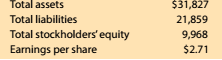Chapter 8, Problem 8.9.3MBA

Chapter
Section
Textbook Problem

Debt and price-earnings ratios Lowe's Companies Inc. (LOW) operates over 1,800 home improvement retail stores and is a competitor of The Home Depot (HD). The following data (in millions) were adapted from a recent financial statement of Lowe's:Compute the ratio of liabilities to Stockholders' equity. Round to one decimal place.

To determine

Concept Introduction:

Debt Ratio:

Debt ratio is the relationship between the Total liabilities and Total Assets of a corporation. Debt ratio shows the part of assets financed by debts. It is calculated by dividing total liabilities by total assets. The formula of debt ratio is as follows:

Debt Ratio = Total LiabilitiesTotal Assets

To Calculate:

The Ratios of Liabilities to Stockholder's Equity

Explanation

The Ratios of Liabilities to Stockholder's Equity is calculated as follows:

 \$ in Millions Stockholder's Equity (A)

Still sussing out bartleby?

Check out a sample textbook solution.

See a sample solution

The Solution to Your Study Problems

Bartleby provides explanations to thousands of textbook problems written by our experts, many with advanced degrees!

Get Started

Find more solutions based on key concepts